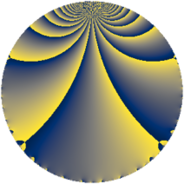# Properties

 Label 126.2.kLevel $126$ Weight $2$ Character orbit 126.k Rep. character $\chi_{126}(17,\cdot)$ Character field $\Q(\zeta_{6})$ Dimension $8$ Newform subspaces $1$ Sturm bound $48$ Trace bound $0$

# Learn more about

## Defining parameters

 Level: $$N$$ $$=$$ $$126 = 2 \cdot 3^{2} \cdot 7$$ Weight: $$k$$ $$=$$ $$2$$ Character orbit: $$[\chi]$$ $$=$$ 126.k (of order $$6$$ and degree $$2$$) Character conductor: $$\operatorname{cond}(\chi)$$ $$=$$ $$21$$ Character field: $$\Q(\zeta_{6})$$ Newform subspaces: $$1$$ Sturm bound: $$48$$ Trace bound: $$0$$

## Dimensions

The following table gives the dimensions of various subspaces of $$M_{2}(126, [\chi])$$.

Total New Old
Modular forms 64 8 56
Cusp forms 32 8 24
Eisenstein series 32 0 32

## Trace form

 $$8q + 4q^{4} + 4q^{7} + O(q^{10})$$ $$8q + 4q^{4} + 4q^{7} + 12q^{10} - 4q^{16} - 24q^{19} - 24q^{22} - 16q^{25} - 4q^{28} - 12q^{31} - 16q^{37} + 12q^{40} + 32q^{43} + 20q^{49} + 12q^{58} + 24q^{61} - 8q^{64} + 40q^{67} - 12q^{70} + 24q^{73} + 28q^{79} + 48q^{82} - 12q^{88} - 24q^{91} - 24q^{94} + O(q^{100})$$

## Decomposition of $$S_{2}^{\mathrm{new}}(126, [\chi])$$ into newform subspaces

Label Dim. $$A$$ Field CM Traces $q$-expansion
$$a_2$$ $$a_3$$ $$a_5$$ $$a_7$$
126.2.k.a $$8$$ $$1.006$$ $$\Q(\zeta_{24})$$ None $$0$$ $$0$$ $$0$$ $$4$$ $$q+(\zeta_{24}-\zeta_{24}^{3})q^{2}+(1-\zeta_{24}^{2})q^{4}+\cdots$$

## Decomposition of $$S_{2}^{\mathrm{old}}(126, [\chi])$$ into lower level spaces

$$S_{2}^{\mathrm{old}}(126, [\chi]) \cong$$ $$S_{2}^{\mathrm{new}}(21, [\chi])$$$$^{\oplus 4}$$$$\oplus$$$$S_{2}^{\mathrm{new}}(42, [\chi])$$$$^{\oplus 2}$$$$\oplus$$$$S_{2}^{\mathrm{new}}(63, [\chi])$$$$^{\oplus 2}$$